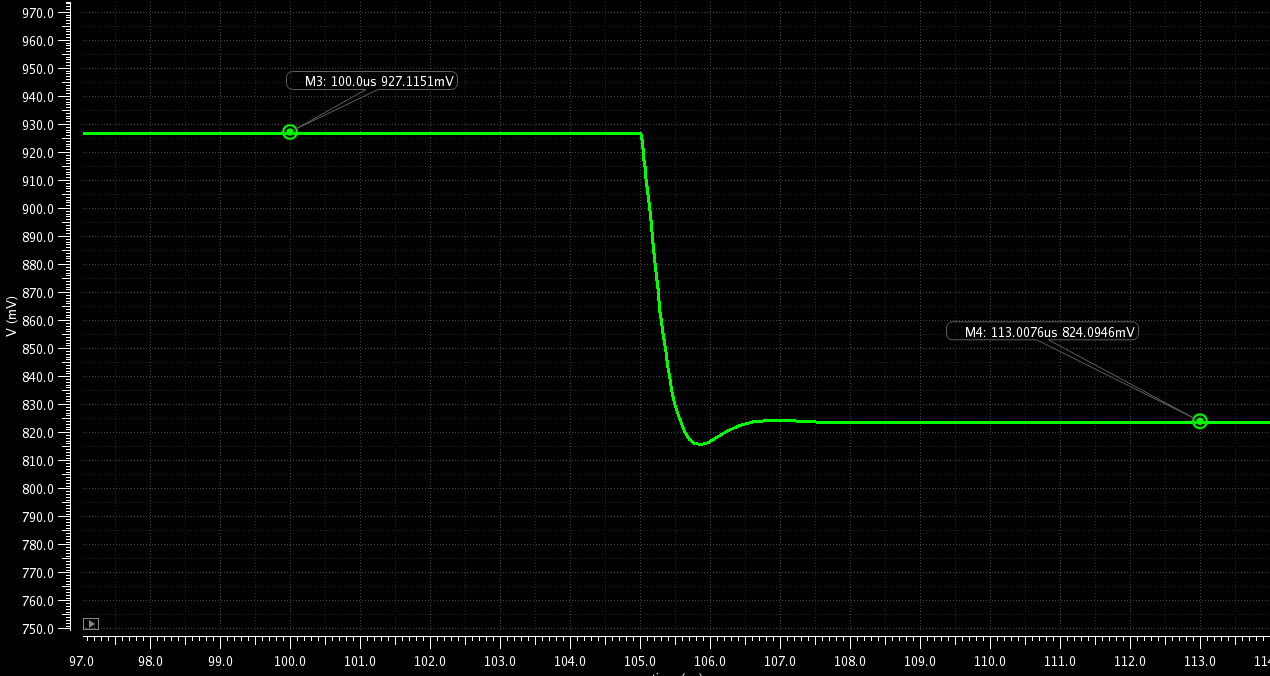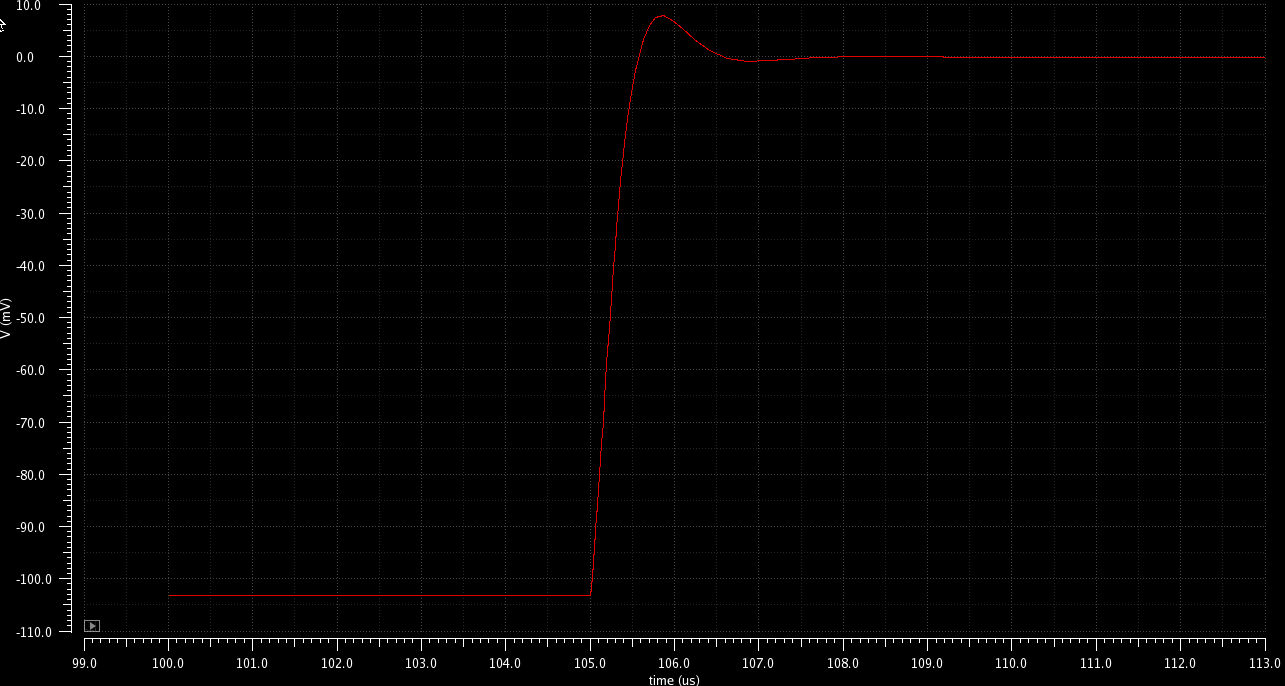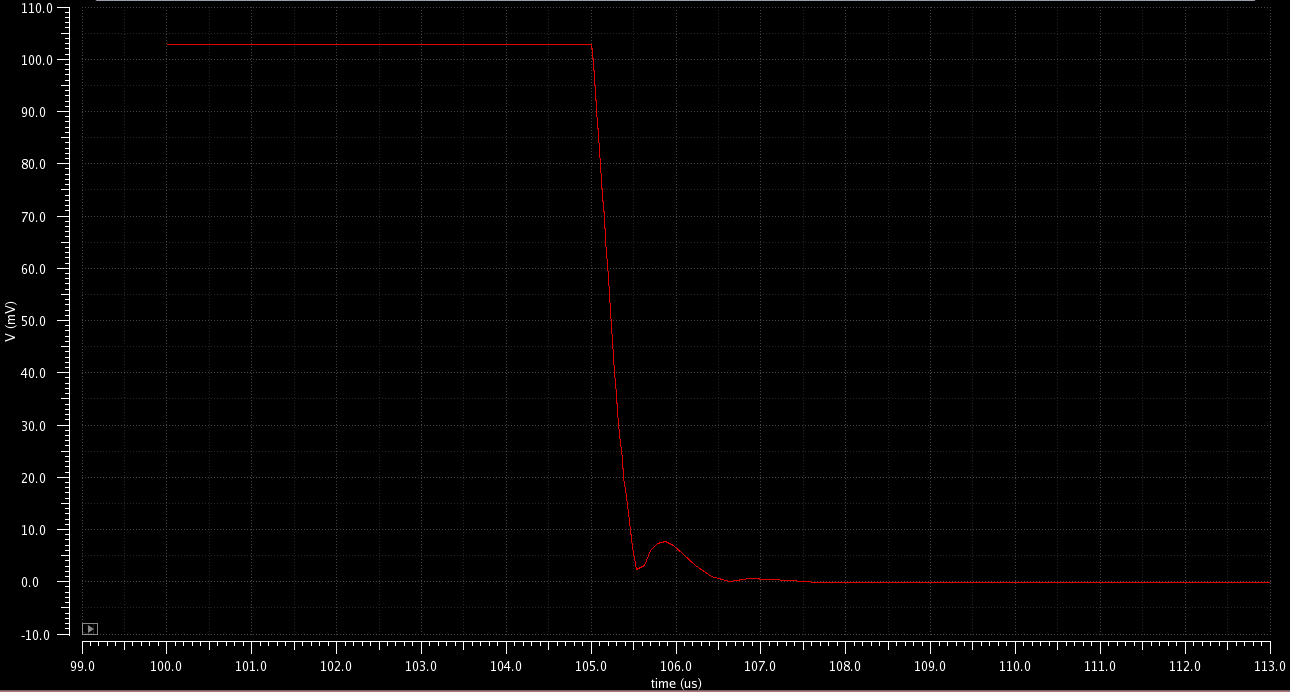# Getting the Settling time in Cadence# Overview [top]

This page describes how you can setup an expression for the settling time without using the Cadence settling time function in the calculator. I have found that the settlingtime function in the calculator is not that reliable and its hard to debug it because I don't know how it operates internally. Here I document a technique I use to calculate the settling time of a waveform using simple functions like value, clip and cross.

# Technique [top]

Let us say we have an event in the wave as shown below:To find the settling time of WAVE1 to P% of final value:
1. Clip the WAVE1 at the extremes of where the settling event occurs. These can be the points M3 (start - 104.5us) and M4 (end - 113us) for the above shown waveform. Make sure only 1 settling event occurs in the clipped waveform and the end point is what the final value you are looking to settle to. The Calculator expression would look something like:
```clip(VT("/WAVE1") 100u 113u)
```
2. Now the initial value is INI (927.115mV) and final value (also the final value of the clip) is FIN (824.095mV). The calculator expressions would look something like:
```value(VT("/WAVE1") 100u) ; INI value
value(VT("/WAVE1") 113u) ; FIN value
```
3. Let WAVE2 = abs(FIN-WAVE1) For the above wave the FIN-WAVE1 looks like:and abs(FIN-WAVE1) looks like:The calculator expression looks something like:
```abs(value(VT("/WAVE1") 113u) - clip(VT("/WAVE1") 100u 113u))
```
4. Now setup a cross function for abs(FIN-WAVE1) to cross the threshold of abs(FIN-INI)*P/100 and measure the last edge (-1 edge number) and the edge can be "either" (instead of rising or falling). This cross function now gives the time point when the signal enters the P% range and settles. The cross function looks like this in the calculator (For P=1%):
```cross( abs(value(VT("/WAVE1") 113u) - clip(VT("/WAVE1") 100u 113u)) abs(value(VT("/WAVE1") 113u) - value(VT("/WAVE1") 100u))*0.01 -1 either nil nil nil)
```
Another threshold could be P% of the absolute final value and not the difference i.e. abs(FIN)*P/100. Depending on what kind of settling you are measuring pick the appropriate threshold.
5. Now subtract the initial stimulus time from this cross function to get the settling time. In the wave shown above the stimulus happenned at 105us. So the result from the last step should subtract 105us to get the settling time. The final expression for the settling time from INI to FIN % settling looks like:
```cross( abs(value(VT("/WAVE1") 113u) - clip(VT("/WAVE1") 100u 113u)) abs(value(VT("/WAVE1") 113u) - value(VT("/WAVE1") 100u))*0.01 -1 either nil nil nil)-105u
```